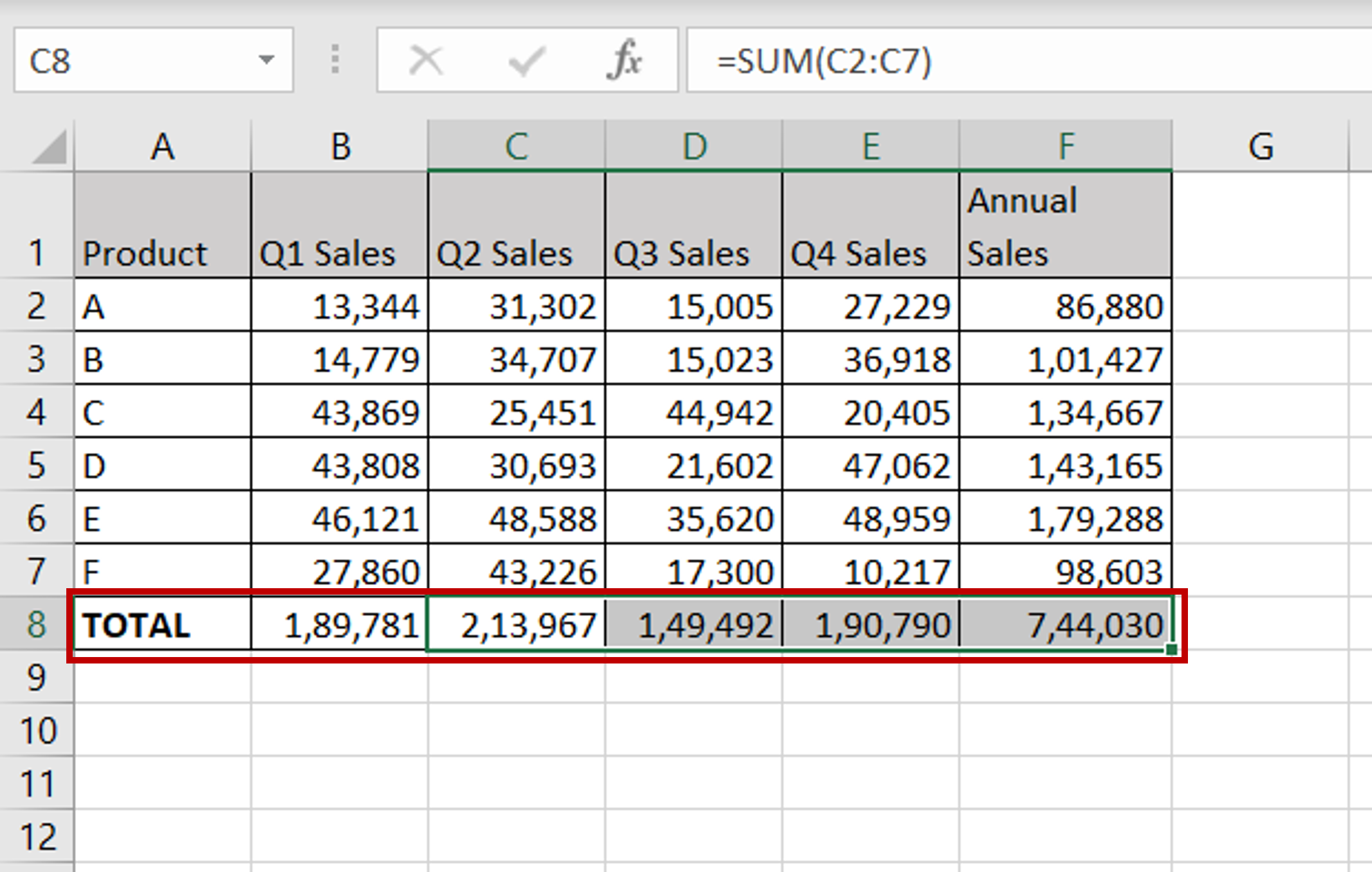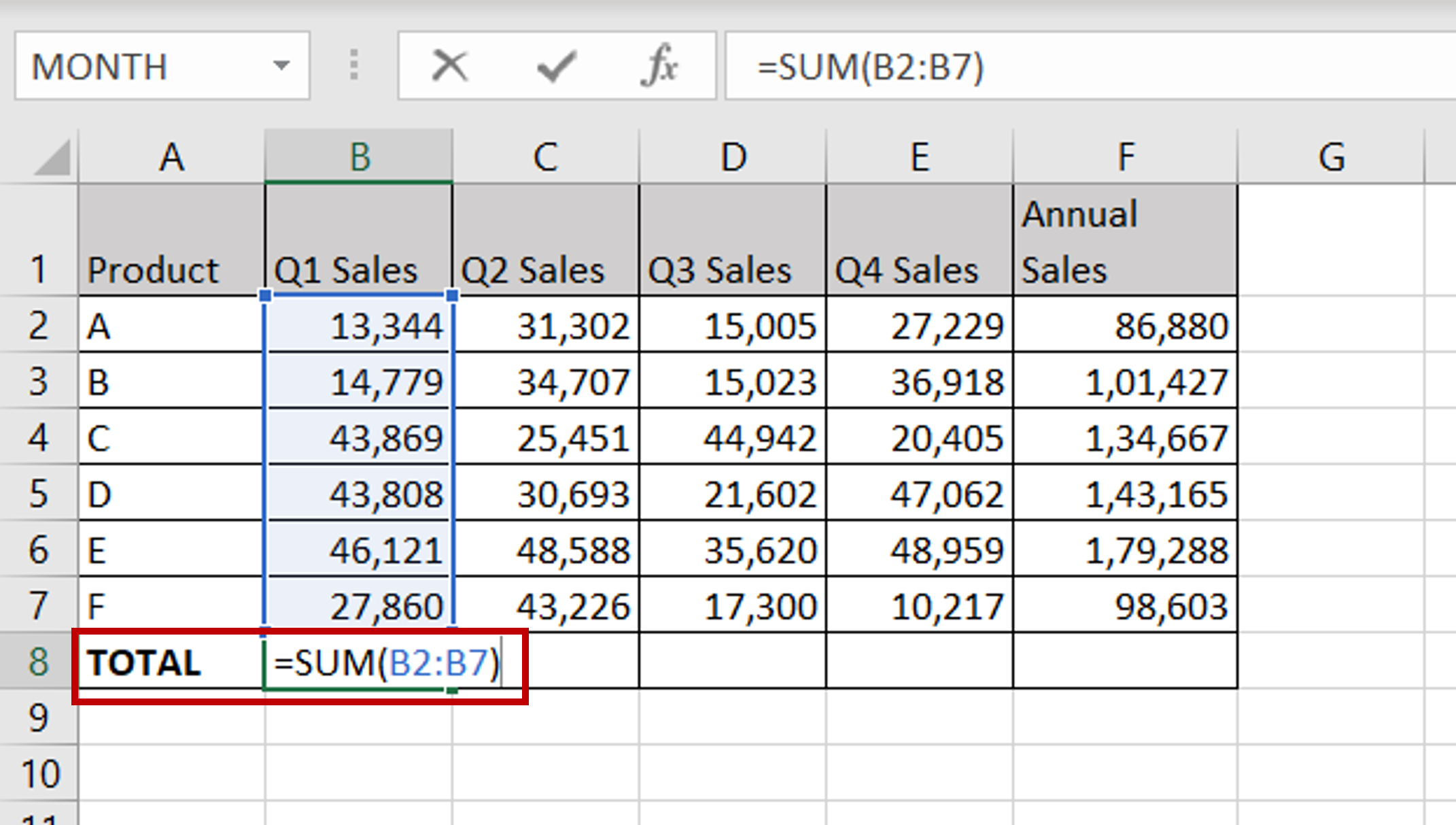# How do I add up a column in ExcelWhen using Excel, you will frequently need to find the totals of columns of numbers. This can be easily done using the SUM function of Excel.

### Step 1 – Use the SUM() function to total the first column– In the destination cell, enter the formula using cell references:
= SUM(Q1 Sales)

### Step 2 – Copy the formula and check the result– Using the fill handle from the first cell, drag the formula to the remaining cells
OR
a) Select the cell with the formula and press Ctrl+C or choose Copy from the context menu (right-click)
b) Select the rest of the cells in the column and press Ctrl+V or choose Paste from the context menu (right-click)
– Check that all columns have been added and that the totals are displayed